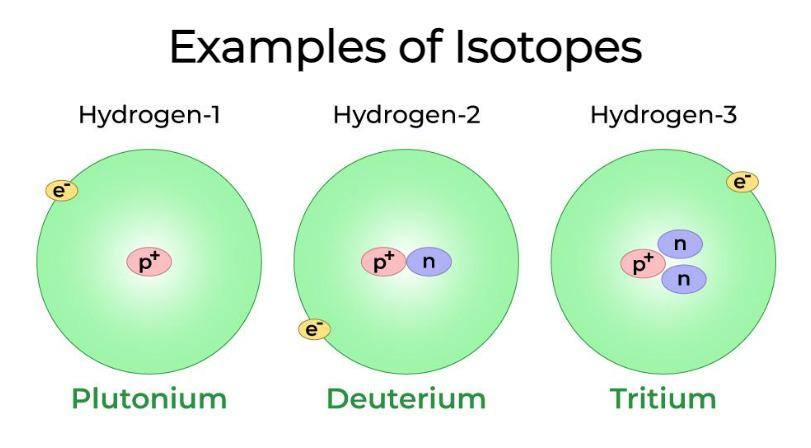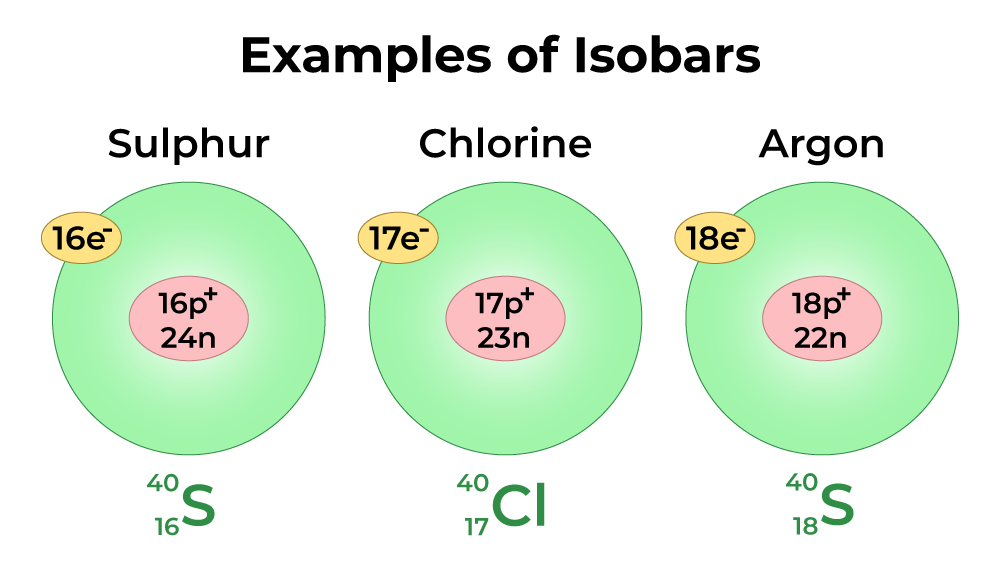GeeksforGeeks App
Open AppBrowser
Continue

## Related Articles

• CBSE Class 11 Chemistry Notes

Isotopes and Isobars are the two important terms that are very often used in atomic structure. Isotopes are elements that have different mass numbers but similar atomic numbers. Similarly, Isotones are elements that have similar mass numbers and different atomic numbers. Thus, the major difference between Isotopes and Isobars is that isotopes belong to the same element while isotones are different elements. Also, isotopes have similar chemical properties. However, isobars have different chemical properties. For example, 12C, 13C, and 14C are the isotopes of Carbon while 26Fe58 and 27Ni58 are Isotones. Let’s understand the difference between Isotopes and Isotones in more detail!

## What are Isotopes?

Isotopes are defined as the elements that have the same atomic numbers but different mass numbers. This implies Isotopes have samenumber of protons and electrons in them.

Hence, the same elements having different atomic structures are called isotopes. As a result, isotopes have identical atomic numbers but various atomic masses. This is because each chemical element has a distinct atomic number because each nucleus contains the same number of protons. However, since the atomic mass is based on the number of neutrons in the nucleus and isotopes have various numbers of neutrons, the atomic masses of isotopes may vary from one another.

### Examples of Isotopes

• Hydrogen: Hydrogen-1 (Protium), Hydrogen-2 (Deuterium), and Hydrogen-3 (Tritium), shown below:• Uranium: Uranium has two naturally occurring isotopes found in Earth’s crust which are Uranium-235 and Uranium-238.
• Carbon: Carbon-12 and carbon-14 are two carbon isotopes and many more.

Let’s understand by the example of isotopes of Carbon, Carbon has 12C, 13C, and 14C as its isotopes mean 12, 13, and 14 are the mass number of Carbon and the atomic number is 6. But as atomic number means the number of protons so all carbon isotopes will have the same number of atoms while the number of neutrons is different.

### Uses of Isotopes

• Goiter (swelling neck) disease can be cured by using an isotope of iodine.
• Treatment of some types of cancer can be done using cobalt isotopes.
• Uranium isotope is used as nuclear fuel.

## What are Isobars?

Isobars are defined as the elements having different atomic numbers but the same mass number. Thus, the elements having different chemical properties but the same physical properties are called isobars.

Chemical elements with the same atomic mass are called isobars. The total amount of protons and neutrons in an element determines its atomic mass. A nucleon is a proton or neutron by itself. As a result, the atomic mass is equal to the number of nucleons. Isobars share the same numbers of nucleons as a result. However, in isobars, the total numbers of protons and neutrons are always different from one another.

The same number of electrons make up each isobar. This suggests that isobars exhibit the same chemical characteristics. Because the atomic masses are the same, the physical characteristics are likewise the same. Isobars are separate chemical elements since their atomic numbers vary.

### Examples of Isobars

• Isobars with atomic mass 64: Cobalt (Co), Nickel (Ni), Copper (Cu), and Iron (Fe).
• Isobars with atomic mass 3: Hydrogen (H) and Helium (He).Let’s understand by the example of isobars with mass number 40: Sulphur (40S16), Chlorine (40Cl17), and Argon (40Ar18). These elements have the same mass number equal to 40 and different atomic numbers varying from 16 to 18 as shown below in the table.

### Uses of Isobars

• Isobars are used in the medical field to treat tumors and blood clots.
• Thyroid disorders can be treated using isobars of iodine.
• Blood cancer can be treated using isobar of phosphorus.

## FAQs on Isotopes and Isobars

Question 1: What are Isotopes and Isobars?

Isotopes are defined as elements that have the same atomic numbers but different mass numbers. However, Isobars are defined as elements having different atomic numbers but the same mass number.

Question 2: Write some examples of Isotopes and Isobars.

Isotopes – Chlorine has two isotopes 35Cl and 37Cl. Hydrogen has 3 isotopes 1H, 2H, and 3H.

Isobars – Carbon and nitrogen have the same mass number 14. Argon and Calcium have the same mass number 40.

Question 3: Write three isotopes of Oxygen and Hydrogen each.

Isotopes of Oxygen are 16O, 17O, and 18O.

Isotopes of Hydrogen are tritium, deuterium, and protium.

Question 4: What is the difference between a stable and unstable isotope?

Stable isotopes are naturally occurring forms of elements that are non-radioactive. Unstable isotopes are atoms having unstable nuclei.

Question 5: What are radioactive isotopes?